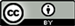1. 引言

2. 研究方法及模型2.1. 事件研究方法

MIT斯隆管理学院学者Kothari等  将事件研究分为短期间事件研究(事件窗小于1年)与长期间事件研究(事件窗大于等于1年)。他们认为短期间事件研究已较成熟，值得信赖，而长期间事件研究弊端明显。因为期间长度越长，整个样本在事件窗内平均异常收益的方差将越大，研究结果变得不太可靠。因此，考虑到短期间事件研究结果可能包含更多信息量和经济含义，所以本文采用短期间事件研究方法，分析结果将充分结合中国钢材交易市场的特点，具有实际应用价值。

2.2. 研究模型

2.2.1. 判断市场定价效率——协整检验模型

ln S S E C t = A 1 ln S S E C t − 1 + ⋯ + A p ln S S E C t − p + B ln S P O T t + ε t (1)

ln S S E C t ' = M 1 ln S S E C t ' − 1 + ⋯ + M l ln S S E C t ' − l + N ln S P O T t ' + K ln S H F E t ' + δ t ' (2a)

ln S H F E t ' = Γ 1 ln S H F E t ' − 1 + ⋯ + Γ q ln S H F E t ' − q + Λ ln S S E C t ' + Φ ln S P O T t ' + η t ' (2b)

2.2.2. 判断市场对新息反应能力——广义主导模型

2.2.3. 研究市场价格发现功能——共同因子模型

3. 市场环境、事件窗口设定及数据说明

4. 研究结果

5. 对结果的讨论

1) 电子交易市场和期货市场均存在着套保、套利空间。在远期交易尚未被压制的时域内，远期价格、

Empirical results of price efficiency, price dominance, and price discovery of Chinese steel market

Γ = 0.10PT = 68.96%PT = 31.04%

Γ = 0.24PT = 86.11%PT = 13.89%

Γ = 0.17PT = 42.88%PT = 57.12%

Γ = 0.12PT = 51.16%PT = 48.84%

Γ = 0.31PT = 33.78%PT = 66.22%

Γ = 0.27PT = 27.47%PT = 30.36%PT = 42.17%
Γ = 0.48

Γ = 0.46PT = 43.14%PT = 43.52%PT = 13.34%
Γ = 0.16

Γ = 0.34PT = 46.81%PT = 4.33%PT = 48.86%
Γ = 0.44

2) 全球金融风暴爆发背景下，电子交易市场在价格主导性和价格发现功能上表现较好，远期价格对新息反应能力较快。金融风暴爆发后，钢材电子交易市场交易量一度上升，亦说明钢铁产业链成员对该市场规避波动风险能力的认可。

3) 钢材期货上市对电子交易市场价格发现功能影响较大。电子交易市场价格发现功能在钢材期货引入后显著变弱，主要原因是电子交易市场在交易成本、流动性、交易量等方面与期货市场存在不小差距。从保证金角度上看，期货交易成本远低于电子交易市场。从流动性方面看，期螺流动性比率高于SSEC热卷板。从交易量看，电子交易市场热卷板主力合约成交量低于期螺纹钢主力合约成交量。当钢材期货市场提高保证金水平后一段时期内，电子交易市场价格发现功能重新得以显现，该市场价格占价格发现比例46.56%，接近50%。

4) 对于保证金水平上调这一行为，电子交易市场和期货市场反应不一。电子交易市场保证金提高前，电子交易市场居主导地位，并且该市场在有效价格形成中贡献份额高达85.56%；保证金水平提高后，电子交易市场对新息反应能力略有下降，但仍具主导性。其价格发现功能有所增强。这表明电子交易市场上调保证金水平这一行为，对电子交易市场价格回归基本面有较大帮助。

5) 市场主导性与市场价格发现功能是两个不同概念，其经济含义不同。常有研究文章将二者混为一谈，认为居主导地位的市场，其价格发现功能也较强。其实不然，本文研究结果显示出二者可能并不一致，它们不是相同的概念。能对密切相关市场同期价格起到引领作用的市场，其价格发现功能可能不是最强的市场。价格“领先–滞后”关系上的结果及相关结论不能代替价格发现功能的研究结论。